## Saturday, February 28, 2009

### The Illusion of Knowledge

Although not along the general theme of this blog, I am obligated to make this post so that I remember this, and hopefully, share it with many other fellow engineers and researchers.

I was deeply motivated and moved by a quote from Daniel J. Boorstin:

The greatest obstacle to discovery is not ignorance, it is the illusion of knowledge.

Indeed, often times we find ourselves locked within an bubble of knowledge, thinking that we know everything and that we have solutions for all, yet, we fail to realize that this only an imaginary crust beyond which lies the unknown.

Cite as:

## Sunday, February 22, 2009

### 8. Derivation of the Continuity Equation in Cylindrical Coordinates

This is one of my favorite derivations. Although it would sound a bit intimidating at first, as none of the standard textbooks carry out the derivation in curvilinear coordinates; it is rather easy to obtain. And guess what? the math is quite rewarding!
So we first have to start by selecting a convenient control volume. The idea here is to pick a volume whose sides are parallel per say to the coordinates. For cylindrical coordinates, one may choose the following control volume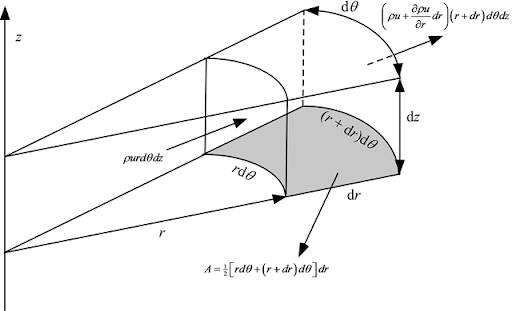Again, as we did in the previous post, we need to account for all the fluid that is accumulating, and flowing through this control volume, namely:
Rate of Rate of Flow In = Accumulation  + Rate of Flow Out
or
Accumulation + Flow Out - Flow In = 0

First, let’s get some basics laid out. The velocity field will be described as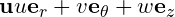I always prefer to use u, v, and w instead of ur, utheta, and uz to save on subscripts, although the latter nomenclature is a bit more descriptive… we’ll get used to it. Now, by construction, the volume of the differential control volume is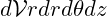while the mass of fluid in the control volume is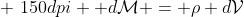The rate of change of mass or accumulation in the control volume is then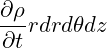For the net flow through the control volume, we deal with it one face at a time. Starting with the r faces, the net inflow is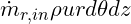while the outflow in the r direction is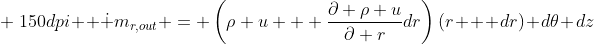So that the net flow in the r direction is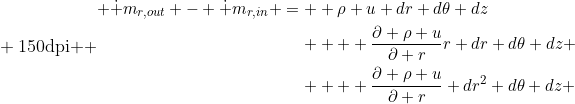Being O(dr^2), the last term in this equation can be dropped so that the net flow on the r faces isThe net flow in the theta direction is slightly easier to compute since the areas of the inflow and outflow faces are the same. At the outset, the net flow in the theta direction is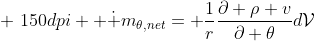We now turn our attention to the z direction. The face area is that of a sector of angle d\theta:

then, the inflow at the lower z face is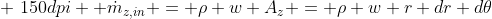while the outflow at the upper z face is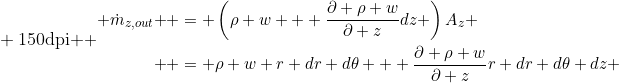Finally, the net flow in the z direction is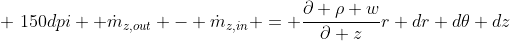Now we can put things together to obtain the continuity equation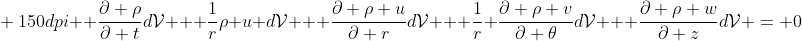dividing by dV and rearranging the r components of the velocity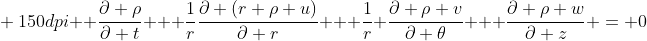Voila!
[Previous: Continuity Eq. in Cartesian Coordinates]

Cite as:
Saad, T. "8. Derivation of the Continuity Equation in Cylindrical Coordinates". Weblog entry from Please Make A Note. https://pleasemakeanote.blogspot.com/2009/02/8-derivation-of-continuity-equation-in.html

## Saturday, February 21, 2009

### 7. Derivation of the Continuity Equation in Cartesian Coordinates

[Previous Article: How Euler Derived the Continuity Equation]
The continuity equation is an expression of a fundamental conservation principle, namely, that of mass conservation. It is a statement that fluid mass is conserved: all fluid particles that flow into any fluid region must flow out. To obtain this equation, we consider a cubical control volume inside a fluid. Mass conservation requires that the the net flow through the control volume is zero. In other words, all fluid that is accumulated inside the control volume (due to compressibility for example) + all fluid that is flowing into the control volume must be equal to the amount of fluid flowing out of the control volume.
Accumulation + Flow In = Flow Out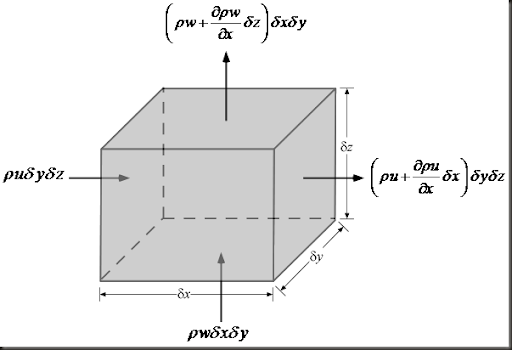The mass of the control volume at some time t isThe time rate of change of mass in the control volume isNow we can compute the net flow through the control volume faces. Starting with the x direction, the net flow isSimilarly, the net flow through the y faces iswhile that through the z faces isUpon adding up the resulting net flow and diving by the volume of the fluid element (i.e. dxdydz), we get the continuity equation in Cartesian coordinatesVoila!

Cite as:
Saad, T. "7. Derivation of the Continuity Equation in Cartesian Coordinates". Weblog entry from Please Make A Note. https://pleasemakeanote.blogspot.com/2009/02/derivation-of-continuity-equation-in.html

## Thursday, February 19, 2009

### The Coming Revolutions in Theoretical Physics

Check out this interesting talk by David Gross

Cite as:

### Windows Defender Update Issues

For some reason, windows defender starts messing up and is not able to detect the latest updates. You will then need to download and install these updates manually. Check this out

How to troubleshoot definition update issues for Windows Defender

I hope that works out for you.

Cite as:

## Tuesday, February 10, 2009

### Enable the Vorticity Magnitude Computation in Fluent Inviscid Simulations

For inviscid models, Fluent does not allow you to compute the vorticity magnitude. While it should usually be available under the velocity section of the variables to plot, an inviscid fluent model lacks this feature. I am not sure why this is the case as inviscid flows are generally rotational! Only irrotational flows have zero vorticity.

So any way, some of my models are run under inviscid flow conditions and to compute the vorticity, one may define a UDF to compute it or simply export the velocity field data and compute it in a data analysis software. I find this rather cumbersome as it cost me a lot of time and the accuracy of my computations may suffer a bit. It could have been just easier if Fluent does that automatically.

Here’s a neat trick: to enable the computation of vorticity in an inviscid Fluent model, simply change your model to laminar and you’ll see that nice vorticity magnitude variable show up!

Go to: Define/Models/Viscous and select Laminar

Voila!

Of course, you should do this after you’re done with your inviscid simulation.

Cite as:
Saad, T. "Enable the Vorticity Magnitude Computation in Fluent Inviscid Simulations". Weblog entry from Please Make A Note. https://pleasemakeanote.blogspot.com/2009/02/enable-vorticity-magnitude-computation.html

## Sunday, February 8, 2009

### Derivation of the Navier Stokes and Fluid Transport Equations

In this series:
1. The Material Derivative
2. The Reynolds Transport Theorem
3. The Continuity Equation
4. The Momentum Equations
1. How Euler Derived the Momentum Equations
2. Cartesian Coordinates
3. Cylindrical Coordinates

Cite as:
Saad, T. "Derivation of the Navier Stokes and Fluid Transport Equations". Weblog entry from Please Make A Note. https://pleasemakeanote.blogspot.com/2008/08/derivation-of-navier-stokes-equations_16.html

### 6. How Euler Derived the Continuity Equation

[Previous Article: The Reynolds Transport Theorem]

I thought that it would be interesting to present Euler’s derivation of the continuity equation for incompressible flows. Although d’Alembert, in 1752, had already presented an equivalent equation in his Essai d’une nouvelle théorie de la résistance des fluides (which he had already submitted to the Academy of Sciences of Berlin in 1749), the one proposed by Euler in 1756 (written 1752) is considered to be the most rigorous.

Euler’s contribution to Fluid Mechanics goes beyond what a scientist may imagine, and was mostly due to four manuscripts published between 1752 and 1755. These are

1. Principia Motus Fluidorum (1756) [pdf]
2. Principes généraux de l’état d’équilibre des fluides (1755) [pdf]
3. Principes généraux du mouvement des fluides (1755) [pdf]
4. Continuation des recherches sur la théorie de mouvement des fluides (1755) [pdf]

The final thing I would like to point out is that Euler’s genius lies partly in his ability to synthesize and introduce world class notation. In this way, he was able to supersede all his predecessors.

Euler starts by saying:

“… I shall posit that the fluid cannot be compressed into a smaller space, and its continuity cannot be interrupted. I stipulate without qualification that, in the course of the motion within the fluid, no empty space is left by the fluid, but it always maintains continuity in this motion…” [Paragraph 6, Principia Motus Fluidorum, Translated by Enlin Pan]

He then argues that if one considers any part of a fluid of this type (i.e. incompressible), then each individual particles fill the same amount of space as they move around. He then infers that if this happens for particles, it should happen to the fluid as a whole (which was his assumption of incompressibility). One is now able to consider an arbitrary fluid element and then track its instantaneous changes ”to determine the new portion of space in which it will be contained after a very small time period”. The amount of space that is occupied by the fluid element after it moves with time should then be equal in size to the old one. This is simply brilliant! Euler says that “this equating of size will fully characterize what can be said about the motion”.

To do this, Euler considers an initial fluid element whose shape is a right triangle. Below is the actual drawing from his manuscript:

Here’s an advanced version of his figure

Figure 2

The ABC triangle is our initial fluid element with an initial area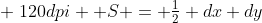Euler then assigns a velocity field at the base vertex A as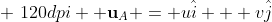Accordingly, the velocities of vertices B and C can be calculated as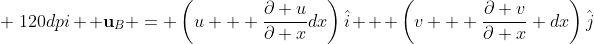andFor a differential time increment, the fluid element ABC will translate, rotate, and stretch while maintaining the same surface area. This is the key to the derivation as mentioned earlier. So the next order of business is to estimate the area of the triangle A’B’C’ and set it equal to that of ABC. if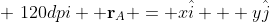then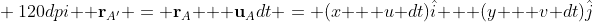subsequently, one can evaluate the position vectors of the remaining verticesNow we have all the required equations to evaluate the area of A’B’C’. For simplicity, we’ll use the cross product. We have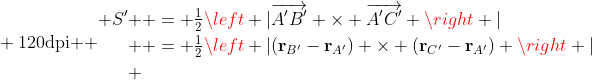Upon a pretty cool evaluation, (which I won’t illustrate here but I still think that you should carry it over because things cancel out very nicely), we obtain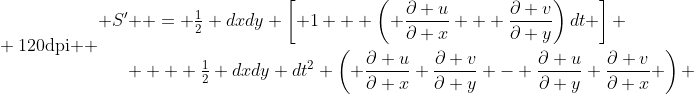Finally, here’s the punch line. By equating S’ and S, we obtain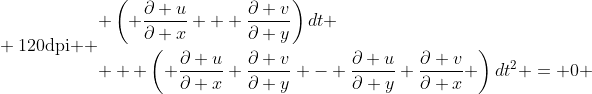or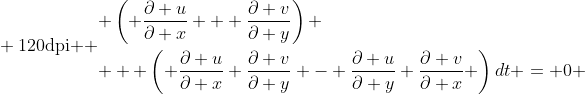Euler then argues that the second term in this last equation is vanishingly small compared to the first term and in the limit of an infinitesimal time increment, we obtain what we call today the continuity equation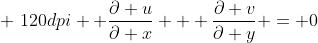Q.E.D

I would like to thank the Euler archive for doing a great service for humanity by archiving Euler’s works as well as providing translations for some of his texts. The quoted paragraphs in orange are taken from Enlin Pan's english translation of Euler’s Principia Motus Fluidorum.

[Previous Article: The Reynolds Transport Theorem]

[Next Article: The Continuity Equation in Cartesian Coordinates]

Cite as:
Saad, T. "6. How Euler Derived the Continuity Equation". Weblog entry from Please Make A Note. https://pleasemakeanote.blogspot.com/2009/02/derivation-of-navier-stokes-equations.html

## Saturday, February 7, 2009

### Setting Column Values in Origin – the Programmers Way!

Do the following
1. Select any of the columns by clicking on its title label
2. Right click and invoke the “Set Values” dialog box
3. Click on the little arrow to the bottom right (“Show Scripts”)
4. In the “Before Formula Scripts” text box, type in the following:
aRef = 10;
aCorrection = 0.01;
// loop over columns – say from column 2 to column 25 – you may need to start at column 2
// since column 1 usually holds the data corresponding to the independent variable

loop(i, 2, 25) {
wcol(i) /= aRef;
wcol(i) += aCorrection;
}
5. Click “Apply” or “OK”

Voila!

Origin is a “professional data analysis and graphing software for sicnetists and engineers”. More often than not, I find myself importing tons of data into worksheets. Most of the time, I would be comparing analytical (yes, some folks on this planet still do analytical work) and numerical data. Without loss of generality, most analytical solutions produce dimensionless data which will eventually end up being compared to numerical or experimental results. Of course, the numerical or experimental results need to be normalized accordingly. Bottom line, you have to go through every column of external data and do what needs to be done: divide, multiply, subtract etc…

In Origin, once you have some values in a column, you can right click on that column and select “Set Column Values” and enter some formula (e.g. Col(A) = Col(A)*10 + 2). The brute force approach is to go over every column, and type in the formula over and over again. So for Column B, you’ll have Col(B) = Col(B)*10 + 2 and so on.

Fortunately, Origin is amazingly modular and allows you to put in your formulas as a script. So here’s the idea: How about writing a script that loops over all the desired columns and sets the values accordingly?

The nice thing about this is that you can use the usual C operations: *=, /=, +=, and –=.

For online video tutorials and goodies related to Origin, check the OriginLab Website.

Enjoy programming!

Update: A couple of graphs made using Origin to illustrate the quality of the resulting graphs. Note that the default graph template used by Origin may look just like an excel graph. You will have to customize your graph to match the look that you are looking for, and of course, reuse the template you have created.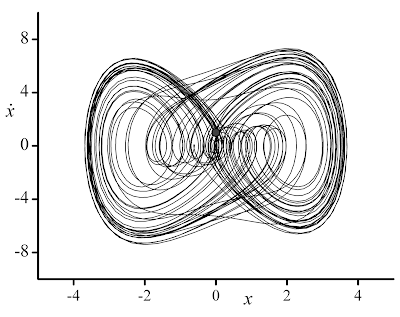Cite as:
Saad, T. "Setting Column Values in Origin – the Programmers Way!". Weblog entry from Please Make A Note. https://pleasemakeanote.blogspot.com/2009/02/setting-column-values-in-origin.html

### LaTeX: Vertical Spacing in Multiline Equations

To add some extra spacing in multiline equation environments (such as align or gather), you can use the \jot command either in the preamble which will affect all your multiline equations, or only locally.

\setlength{\jot}{height}

Here’s an example

\begin{align*}
\setlength{\jot}{12pt}
a + c^3 & = 0 \\
a + b^2 & = 0 \\
d + c^{-1} & = 10 \\
d + a^2 & = \pi
\end{align*}

it looks like this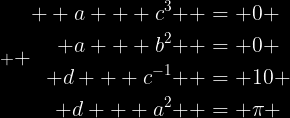Voila!

ref: LaTeX Community

Cite as:

## Friday, February 6, 2009

### LaTeX: Undefined Control Sequence \printglossary

This happens when updating to the latest version of MiKTeX. Assuming that you are including the “nomencl” package, then you need to do the following substitutions:

1. Replace \makeglossary with \makenomenclature
2. Replace \printglossary with \printnomenclature

Voila!

ref: Somacon

The nomencl package is useful for producing nomenclatures/glossaries. For more information check the nomencl package documentation @ the University of Cambridge [this is a pdf file].

Cite as:

### Install Google Desktop on Vista 64 bit

Do the following:

2. Start a command prompt
3. Browse to the directory where you have saved the downloaded file.
(If you are not familiar with MS-Dos @ all, then you can download the file and store it on the root of your hard drive (i.e. C:\), then in the command prompt, simply type in
cd C:\

Voila!

Cite as: